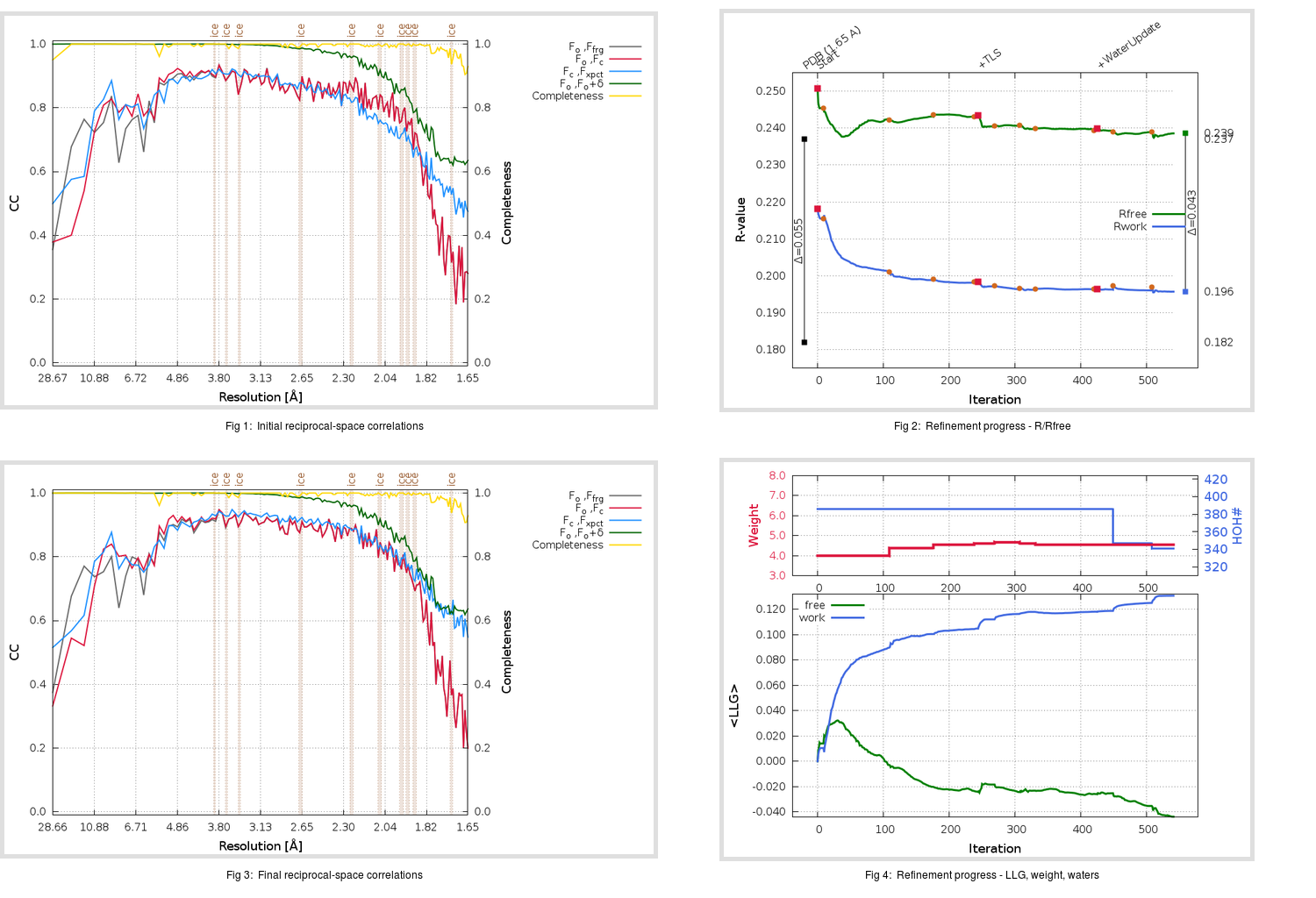Content:

```    Diffraction limits & principal axes of ellipsoid fitted to diffraction cut-off surface:
2.003         0.9961   0.0000  -0.0882       0.993 a* - 0.120 c*
1.721         0.0000   1.0000   0.0000       b*
1.692         0.0882   0.0000   0.9961       0.229 a* + 0.973 c*
```

## Deposited

` `
 Date deposited Date data collection Resolution R, Rfree 20200303 20200226 1.65 0.1790 0.2370

Molprobity (CCP4 7.0 version) summary:

```Ramachandran outliers =   0.33 %
favored =  99.01 %
Rotamer outliers      =   1.14 %
C-beta deviations     =     1
Clashscore            =   6.77
RMS(bonds)            =   0.0145
RMS(angles)           =   1.85
MolProbity score      =   1.42
Resolution            =   1.65
R-work                =   0.1790
R-free                =   0.2370
```

```Number of waters      =   386

<B> (all atoms) =   24.18 ( sd =    8.22 ) for       2779 non-hydrogen atoms
<B>   (protein) =   23.54 ( sd =    7.27 ) for       2363 non-hydrogen atoms
<B>     (water) =   29.32 ( sd =   15.44 ) for        386 non-hydrogen atoms
<B>    (others) =   44.00 ( sd =   23.65 ) for         30 non-hydrogen atoms

B min/max       (all non-hydrogen atoms) =    3.24 /   73.76
B min/max   (protein non-hydrogen atoms) =   12.82 /   65.02
B min/max     (water non-hydrogen atoms) =    3.24 /   73.76
B min/max     (other non-hydrogen atoms) =   23.00 /   69.32
```

## BUSTER (re-)refinement

` `

Molprobity (CCP4 7.0 version) summary:

```Ramachandran outliers =   0.33 %
favored =  98.34 %
Rotamer outliers      =   0.38 %
C-beta deviations     =     0
Clashscore            =   3.39
RMS(bonds)            =   0.0116
RMS(angles)           =   1.56
MolProbity score      =   1.13
Resolution            =   1.65
R-work                =   0.1957
R-free                =   0.2386
```

```Number of waters      =   341

<B> (all atoms) =   25.29 ( sd =    8.39 ) for       2734 non-hydrogen atoms
<B>   (protein) =   24.74 ( sd =    7.11 ) for       2363 non-hydrogen atoms
<B>     (water) =   30.71 ( sd =   17.88 ) for        341 non-hydrogen atoms
<B>    (others) =   33.12 ( sd =   14.04 ) for         30 non-hydrogen atoms

B min/max       (all non-hydrogen atoms) =    5.41 /  134.59
B min/max   (protein non-hydrogen atoms) =   14.68 /   62.60
B min/max     (water non-hydrogen atoms) =    5.41 /  134.59
B min/max     (other non-hydrogen atoms) =   18.03 /   41.16
```

Refinement progression:Results:

` `
 File Remark 5R7Y_aB_refine.01_03_refine.pdb.gz exact refinement commands are in header 5R7Y_aB_refine.01_03_refine.mtz.gz including original deposited data and several re-refinement map coefficients 5R7Y_aB_refine.01_03_BUSTER_model.cif.gz including any non-standard compound restraints 5R7Y_aB_refine.01_03_BUSTER_refln.cif.gz